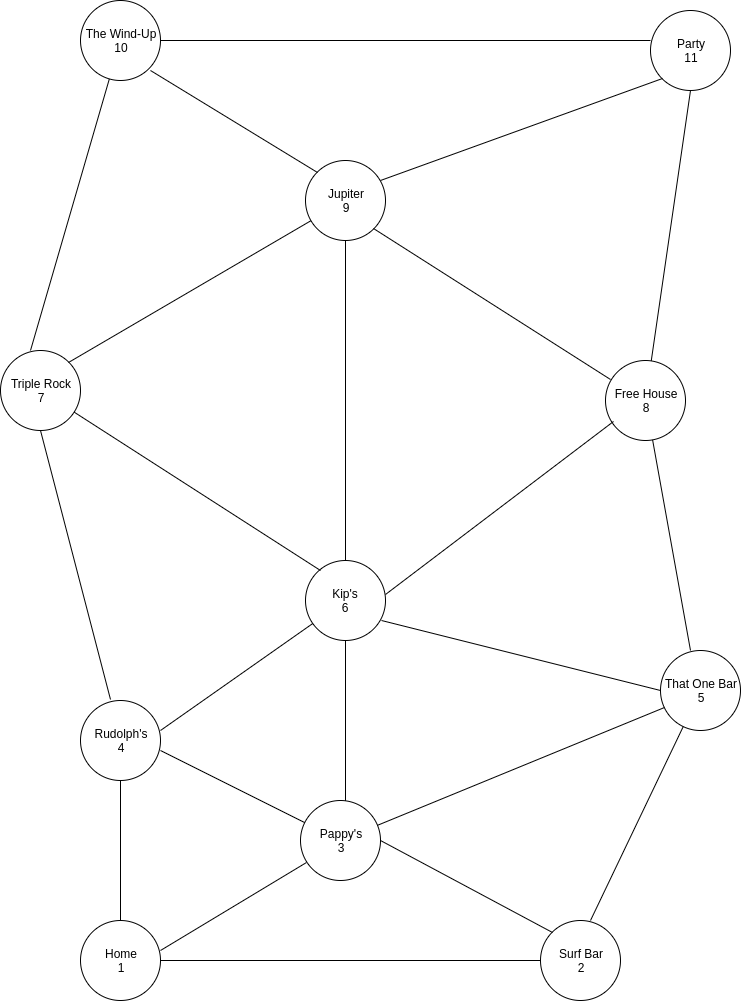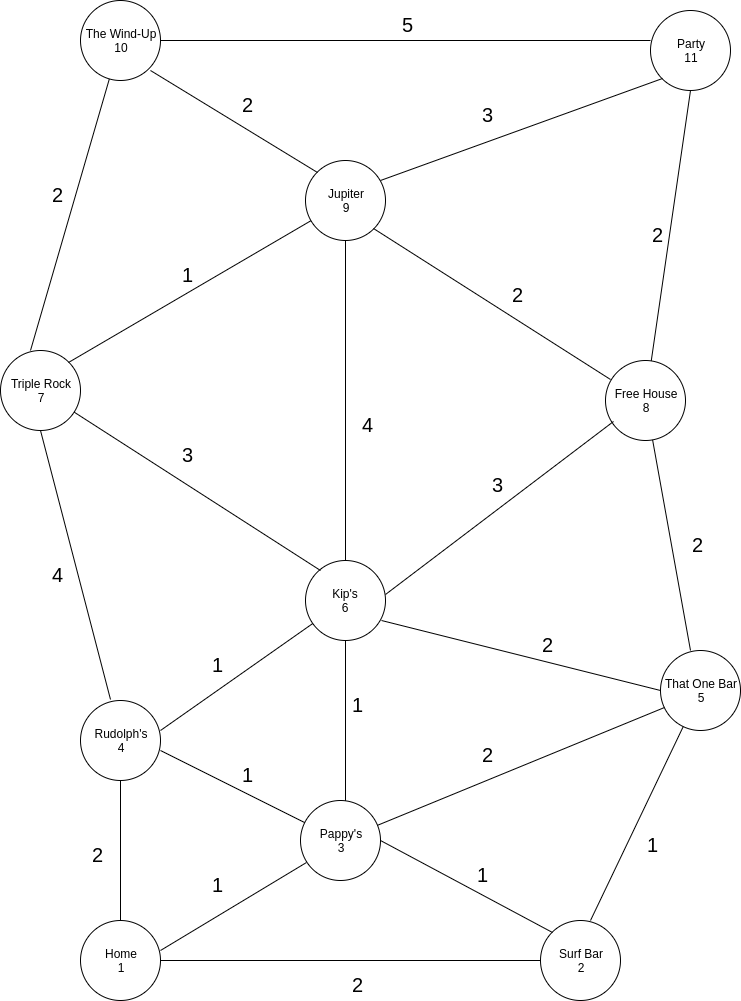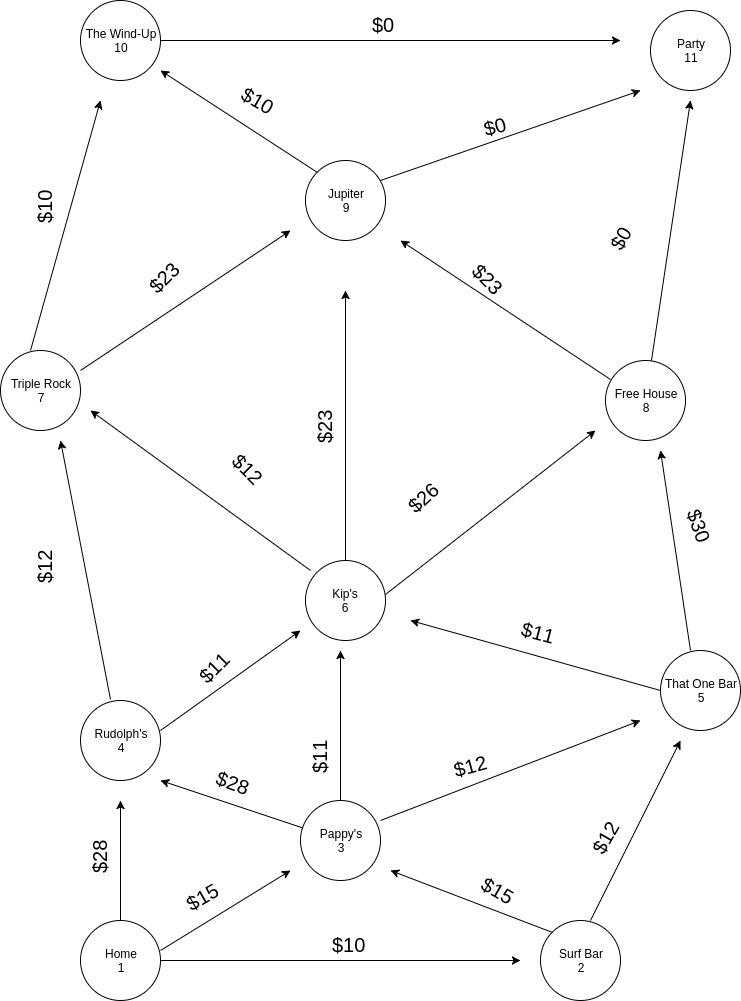Dijkstra’s algorithm was perhaps the first algorithm that inspired me to pursue further self-study into algorithms, algorithm design, and algorithm analysis. Why? When I first discoverd it and attempted to understand it, the algorithm was frankly a little intimidating and somewhat ungraspable. But I was rather hasty and impatient in this initial pursuit. I approached it again and took the time to dissect it, and what I discoverd was that the meat of the logic behind the algorithm wasn't complicated at all -- and what was once intimidating collapsed into a beautifully simple pathfinding procedure that produces the shortest paths from a source node to every other node in a weighted graph

Dijkstra's algorithm also helped to develop my appreciation and understanding of graphs, which is fundamental in understanding this algorithm. So, I open this post going over the basic background terms and concepts in graph theory that Dijkstra's algorithm relies on.

## Background

A graph is a data structure that models relationships between nodes, or vertices, which themselves often represent objects. A common, and effective, analogy for understanding graphs is a map. Take, for example, a map of bars between my home and a party my friend and I have to get to where each node within the graph represents either a bar, my home, or the party we have to get to.Edges connect nodes to one another, as illustrated through the web of lines between the nodes above. Pappy's (node 3) and Kip's (node 6) share an edge, for example, so these two nodes have a relationship with one another. The significance of that relationship is undefined at the moment, as the graph above is unweighted, but nonetheless, there is still a relationship between the two nodes.

The graph above can be more formally denoted as G = (V, E), where graph G is an ordered pair consisting of a set of verticies, here denoted by V, and a set of edges, here denoted by E.

Edges in a graph can be attributed with a value, or a weight.Illustrated above is a weighted, undirected graph because although the edges are ascribed with a value -- or, a weight -- which is the number of miles between bars, there are no directions to the edges, so navigation between connected nodes goes both ways and the weight is applicable in both directions.

In this case, moving between Pappy's and Kip's is 1 mile, whether I'm going to Kip's from Pappy's or from Kip's to Pappy's.

More formally, the graph above is denoted as G2 = (V2, E2). It contains the edge (Pappy's, Kip's) ∈ E2, which means that the ordered pair (Pappy's, Kip's) is an element of the set E2. The graph G2 = (V2, E2) is then understood to be undirected because edge (Pappy's, Kip's) ∈ E2 implies that edge (Kip's, Pappy's) ∈ E2.

Using Dijkstra’s algorithm, I could figure out the most mile-efficient route I could take to our destination, the party my friend and I have to get to, stopping at each bar on the way. But that has little relevance to us at this moment. My buddy and I are more interested in getting a little buzz before the party with as little money as possible and only stopping at a bar for a drink each. So, I do some research and calculate how much it would cost for 2 drinks at each bar within the map.Illustrated above is a graph G3 = (V3, E3), and it is understood to be both weighted because the edges have a cost and directed because edge (Home, Pappy's) ∈ E3 does not imply that (Pappy's, Home) is also in E3. In fact, (Pappy's, Home) ∉ E3.

With direction attached to the edges in G3, navigation between connected nodes are restricted by the edge's direction. For example, there are three edges connected to my Home (node 1), and they only extend out. So, I can only move outwards from Home, the source node, to either Rudolph's, Pappy's, or Surf Bar, the destination nodes in this relationship. I cannot navigate back home, since there are no directed edges pointing to it.

The weight of the edges above represents how much it would cost to get two drinks at the destination node. So, the edge connecting Kip's, the source node, to Triple Rock, the destination node, has a weight of \$12, which means that I can get 2 drinks at Triple Rock for \$12. This is also illustrated in the edge connecting Rudolph's to Triple Rock.

With this background information, let’s see how Dijkstra’s algorithm can be used produce the most cost-efficient route to the party from my home, so that my buddy and I can stop at a few bars along the way and get a decent buzz without spending a lot of money.

## Understanding Dijkstra's Algorithm

While there are a number of variations of Dijkstra’s algorithm, this discussion will focus on a variation that implements a priority queue, specifically a minimum priority queue.

A priority queue is basically a queue that organizes the elements within it based on a priority. It's a useful data structure that supports the basic operations of insert, find minimum (or maximum), and delete minimum (or maximum). In this case, an element with the highest priority will have the lowest value, making it a min-priority queue, so at any given time, and assuming the priority queue has more than a single element inside it, the element with the lowest value within the collection will be at the front of the queue.

To summarize the workings of the min-priority queue implementation of Dijkstra’s algorithm, the algorithm works by keeping track of the lowest value for the path weight to a given node from the source node, updating the path weight to a given node when a lower value is found.

The algorithm does some initial setup work by placing every node within the graph into the priority queue, setting each node with a default of infinity, or the maximum value for an Integer, and creating an adjacency set, which contains mappings between a node and a set of its edges. This is followed by setting the path weight of the starting node to 0, bringing it to the front of the priority queue.

After this setup the meat of the algorithm is as follows:

1) While there are nodes in the priority queue, 2) dequeue a node, 3a) and loop through the set of edges for the dequeued node.

3a) For each destination node in the set of edges for the dequeued node, 3b) compare the stored path value for the destination node with the path value for the dequeued node added with the weight of the edge connecting the dequeued node to the destination node. If the currently stored path value for the destination node is larger, then we know we found a shorter path to it via the dequeued node, 4) so we update the path value for the destination node as the sum of the path value for the dequeued node and the weight of the edge connecting it to the destination node.

Updating a node’s path value will cause the priority queue to reorganize, shifting nodes, so that the node with the smallest path value is at the front of the queue.

These operations continue until the priority queue is empty: so, to reiterate, the node at the front of the queue is removed, its set of edges are looped over and the destination node of these edges are updated if necessary, and the priority queue is reorganized.

When the algorithm is finished, the weight of the shortest path to each node from the source node will be produced.

## Coding Dijkstra's Algorithm

I structured my implementation of Dijsktra’s algorithm to accept input in the following format, which is read from a text file. This format, by the way, is identical to the HackerRank challenge found and read here:

```1
11 22
1 2 10
1 3 15
1 4 28
2 3 15
2 5 12
3 4 28
3 6 11
3 5 12
4 6 11
4 7 12
5 6 11
5 8 30
6 7 12
6 9 23
6 8 26
7 9 23
7 10 10
8 9 23
8 11 0
9 10 10
9 11 0
10 11 0
1
```

The algorithm’s output is also identical to the format of the HackerRank challenge:

```10 15 28 22 26 38 52 49 48 48
```

Here is the code, written in Java, which just contains the meat of the min-priority queue implementation of Dijkstra's algorithm:

``````Scanner in;

try {
in = new Scanner(new File("blogTest.txt"));
int numberOfCases = in.nextInt();

for (int t = 0; t < numberOfCases; t++) {
int numberOfNodes = in.nextInt();
int numberOfEdges = in.nextInt();

node[] nodes = new node[numberOfNodes];
PriorityQueue<node> queueOfNodes = new PriorityQueue<node>(numberOfNodes, new NodeComparator());
HashMap<node, List<Edge>> adjacencySet = new HashMap<node, List<Edge>>();

for (int n = numberOfNodes; n > 0; n--) {
node newNode = new node(n);

nodes[n - 1] = newNode;
}

for (int a1 = 0; a1 < numberOfEdges; a1++) {
int source = in.nextInt();
int destination = in.nextInt();
int weight = in.nextInt();

node sourceNode = nodes[source - 1];

//If an undirected graph, be sure to
}

int startingNodeNumber = in.nextInt();
node startingNode = nodes[startingNodeNumber - 1];
startingNode.updatePathWeightTo(0);

//the Java implementation of the priority queue does not
//reorder itself when an element is updated,
//so the reordering is forced by removing the node and re-adding it,
//but this adds to the time efficiency,
//as the Java implementation of remove is an O(n) operation
queueOfNodes.remove(startingNode);

//Beginning of the meat of the algorithm:
while(!queueOfNodes.isEmpty()) {
node currentNode = queueOfNodes.remove();

node destinationNode = nodes[e.destination - 1];

if(destinationNode.getWeightTo() > currentNode.getWeightTo() + e.weight) {

destinationNode.updatePathWeightTo(currentNode.getWeightTo() + e.weight);
destinationNode.updatePreviousNodeTo(currentNode.element);

if(queueOfNodes.removeIf(i -> i.element == destinationNode.element)) {
};
}
}
}
//End

String results = "";
for (int i = 1; i <= numberOfNodes; i++) {
if (i == startingNodeNumber) { continue; }
node iNode = nodes[i - 1];
results += ((iNode.getWeightTo() == Integer.MAX_VALUE ? -1 :  iNode.getWeightTo() + " "));
}

System.out.println(results);
}
}
catch (Exception ex) {
System.out.println("Ex: " + ex);
}

``````

## Analysis of Dijkstra's AlgorithmThrough my analysis, the meat of this Java implementation of Dijkstra's algorithm runs in O(V2E) time where V is equal to the cardinality of the set of verticies and E is equal to the cardinality of the set of edges in the graph.

This isn't the best implementation of Dijkstra's algorithm, and its inefficiency draws primarily from the underlying implementation of the priority queue in Java. The biggest cause for this inefficiency stems from the Java priority queue not reordering itself when one of its elements is updated, which in this case happens when the path to a node is updated. So the reordering is forced by explictly removing the updated node from the priority queue which is a linear operation because the updated node may or may not be at the front of the queue. The updated node is then re-added to the priority queue in logarithmic, reordering it in the underlying heap of the Java priority queue. Despite this, this implementation is useful for understanding the meat of the logic in Dijkstra's algorithm while using the Java tools available. With a thorough understanding of the logic of the algorithm, you can then move forward with a more efficient implementation.

If there are any errors in my analysis, please feel free to point them out. I'd be grateful for the review.